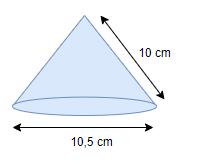# NCERT solutions for class 9 maths chapter 13 exercise 13.3

In this page we have ncert solutions for class 9 maths chapter 13 for EXERCISE 13.3 . Hope you like them and do not forget to like , social share and comment at the end of the page.

## Chapter 13 Ex 13.3

We are assuming the value of π=22/7 in all the solutions
Question 1
Diameter of the base of a cone is 10.5 cm and its slant height is 10 cm. Find its curved surface area.Solution
Diameter of the base of a cone =d=10.5 cm
Radius of the base of a cone =r=5.25 cm
Slant height of the cone =l=10 cm
Curved Surface Area of the cone =π.r.l= (22/7)×5.25×10=165 cm2

Question 2
Find the total surface area of a cone, if its slant height is 21 m and diameter of its base is 24 m?
Solution
Radius (r) of the base of cone = 12 m
Slant height (l) of cone = 21 m
Total surface area of cone = πr(r + l) =1244.57  m2-

Question 3
Curved surface area of a cone is 308 cm2 and its slant height is 14 cm. Find radius of the base  And total surface area of the cone. Solution
CSA =308 cm2
Slant height =14cm
Now CSA =πrl
308=(22/7)X14Xr
R=7 cm
Total surface area
=πrl+πr2
=462 cm2

Question 4
A conical tent is 10 m high and the radius of its base is 24 m. Find
(i)Slant height of the tent
(ii)Cost of the canvas required to make the tent, if the cost of 1 m2 canvas is Rs 70. Solution
Height of the conical tent =h=10 m
Radius of the base of conical tent =r=24 m
Slant height of the conical tent = l = Curved Surface Area of the tent =π.r.l=(22/7)×24×26  m2
Cost of canvas to make the tent @Rs 70 per 1 m2
=70×(22/7)×24×26= Rs 137280

Question 5
What length of tarpaulin 3 m wide will be required to make conical tent of height 8 m and base radius 6 m? Assume that the extra length of material that will be required for stitching margins and wastage in cutting is approximately 20 cm. [Use π = 3.14]
Question 6
The slant height and base diameter of a conical tomb are 25 m and 14 m respectively. Find the cost of white-washing its curved surface at the rate of Rs 210 per 100 m2. Solution
Slant height (l) of conical tomb = 25 m
Base radius (r) of tomb =  7 m
CSA of conical tomb = πrl
= 550 m2
Cost of white-washing 100 m2 area = Rs 210
Cost of white-washing 550 m2- area =Rs (210/100) X550
= Rs 1155
Therefore, it will cost Rs 1155 while white-washing such a conical tomb.

Question 7
A joker’s cap is in the form of right circular cone of base radius 7 cm and height 24 cm. Find the area of the sheet required to make 10 such caps. Solution
Base radius of conical cap =r=7 cm
Height of conical cap =h=24 cm
Slant height of conical cap = l = l = Curved Surface area of 1 cap = Area of the sheet required to make 1 cap =π.r.l
=(22/7)×7×25=550 cm2

Question 8
A bus stop is barricaded from the remaining part of the road, by using 50 hollow cones made of recycled cardboard. Each cone has a base diameter of 40 cm and height 1 m. If the outer side of each of the cones is to be painted and the cost of painting is Rs 12 per m2, what will be the cost of painting all these cones? (Use π = 3.14 and take √1.04 =  1.02)..
Solution
Radius of the cone =r=40/2 cm=.2 m
Height of the cone =h=1 m
Slant height of cone = l = l = Curved surface of cone=πrl=.64056 m2
Curved surface area of 50cone=50X .64056 m2
Now cost of painting 1m2 =R12
Then cost of painting 50 cone will be =
=12X50X .64056
=Rs 384.556

## Summary

1. NCERT solutions for class 9 maths chapter 13 Ex 13.3 has been prepared by Expert with utmost care. If you find any mistake.Please do provide feedback on mail. You can download the solutions as PDF in the below Link also
2. This chapter 13 has total 8 Exercise 13.1 ,13.2 ,13.3,13.4,13.5,13.6,13.7and 13.8. This is the third exercise in the chapter.